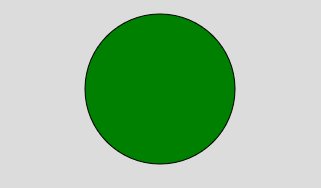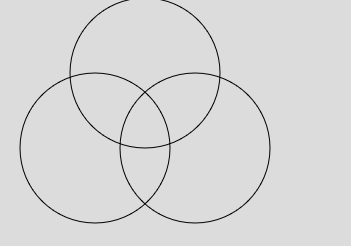# p5.js | circle() Function

• Last Updated : 24 Jul, 2019

The circle() function is used to draw the circle on the screen. A circle is the closed shape. A circle can be created by using the center and radius of the circle.

Syntax:

`circle(x, y, d)`

Parameters:

• x: It is used to set the x-coordinate of the center of the circle.
• y: It is used to set the y-coordinate of the center of the circle.
• d: It is used to set the diameter of the circle.

Example 1:

 `function` `setup() {  ` `     `  `    ``// Create Canvas of given size  ` `    ``createCanvas(400, 300);  ` ` `  `}  ` ` `  `function` `draw() {  ` `     `  `    ``background(220); ` `     `  `    ``// Use color() function ` `    ``let c = color(``'green'``); ` ` `  `    ``// Use fill() function to fill color ` `    ``fill(c); ` `   `  `    ``// Draw a circle  ` `    ``circle(200, 100, 150);  ` `   `  `} `

Output:Example 2:

 `function` `setup() {  ` `     `  `    ``// Create Canvas of given size  ` `    ``createCanvas(400, 300);  ` ` `  `}  ` ` `  `function` `draw() {  ` `     `  `    ``background(220); ` `   `  `    ``// Draw a circle  ` `    ``circle(200, 150, 150);  ` `   `  `    ``noFill(); ` `   `  `    ``// Draw a circle  ` `    ``circle(100, 150, 150);  ` `   `  `    ``// Draw a circle  ` `    ``circle(150, 75, 150); ` `   `  `} `

Output:Reference: https://p5js.org/reference/#/p5/circle

My Personal Notes arrow_drop_up
Recommended Articles
Page :